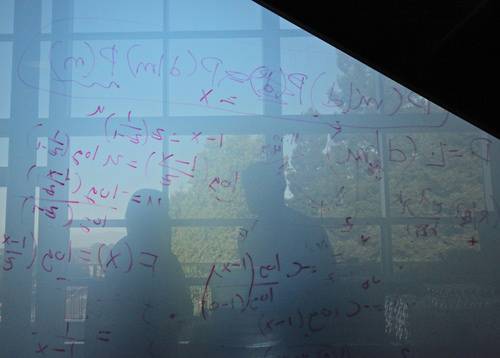# 1.7.4: Simplify Algebraic Expressions

•• Contributed by CK12
• CK12

## Simplify Polynomials by Combining Like TermsFigure $$\PageIndex{1}$$

Jessie is stuck on her math homework. She is stuck on the problem 5x−3y−9x+7y. The directions are asking her to simplify, but she isn’t sure how to do that. Do you know?

In this concept, you will learn to simplify polynomials by combining like terms.

### Combining Like Terms

A polynomial is an algebraic expression that shows the sum of monomials. Since the prefix 'mono' means 'one', a monomial is a single piece or term. The prefix 'poly' means 'many'. So the word polynomial refers to multiple terms in an expression. The relationships between the terms may be sums or differences.

Polynomial expressions include:

• x2+5
• 3x−8+4x5
• -7a2+9b−4b3+6

You can simplify polynomials by combining like terms. In mathematics, you are able to combine like terms but you cannot combine unlike terms.

Terms are considered like terms if they have exactly the same variables with exactly the same exponents.

A term can also be a single number like 7 or -5. These are called constants.

Any term with a variable has a numerical factor called the coefficient. The coefficient of 4x is 4. The coefficient of -7a2 is -7. The coefficient of y is 1 (because its numerical factor is an unwritten number 1. You could write “1y” to show that the coefficient of y is 1 but it is not necessary because any number multiplied by 1 is unchanged).

Here are some examples of like and unlike terms:

7n and 5n are like terms because they both have the variable n with an exponent of 1.

4n2 and -3n are not like terms because, although they both have the variable n, they do not have the same exponent.

5x3 and 8y3 are not like terms because, although they both have the same exponent, they do not have the same variable.

Like terms can be combined by adding their coefficients.

7n+5n=12n

3x3+5x3=8x3

−2t4−10t4=-12t4

2n2−3n+5n2+11n=7n2+8n

Notice that the exponent does not change when you combine like terms. If you think of 7n as simply a shorter way of writing n+n+n+n+n+n+n and 5n as a shorter way of writing n+n+n+n+n, then combining those like terms would result in (n+n+n+n+n+n+n)+(n+n+n+n+n), which is the same as 12n. So 7n+5n=12n.

### Examples

Example $$\PageIndex{1}$$

Here is the problem that Jessie is stuck on:

5x−3y−9x+7y

Solution

First, consider the like terms and combine them:

5x−9x-3y+7y==-4x+4y

Then write the terms in a single expression again:

-4x+4y

Example $$\PageIndex{1}$$

Simplify by combining like terms:

15x−12x+3y−8x+7y−1+5

Solution

First, let’s look at the like terms and combine them.

15x−12x−8x=-5x

3y+7y=10y

-1+5=4

Then rewrite the combined terms in a single expression:

-5x+10y+4

Example $$\PageIndex{1}$$

Simplify by combining like terms.

2x−8y−4x+7y+9

Solution

First, let’s look at the like terms and combine them where possible.

2x−4x=-2x

-8y+7y=-y

9=9

Then rewrite the terms in a single expression:

-2x−y+9

Example $$\PageIndex{1}$$

Simplify by combining like terms.

5a+3b−8b+a−7

Solution

First, let’s look at the like terms and combine them.

5a+a=6a

3b−8b=-5b

-7=-7

Then rewrite the terms in a single expression:

6a−5b−7

Example $$\PageIndex{1}$$

Simplify by combining like terms.

5a−7b=8b−2a+8a−9+8

Solution

First, look at the like terms and combine them.

5a−2a+8a=11a

-7b+8b=b

-9+8=-1

Then rewrite the combined terms in a single expression:

11a+b−1

### Review

Simplify the following polynomials by combining like terms.

1. 6x+7−18x+4
2. 5x−7x+5x+4−9
3. 3x+8y−5x+3y
4. 17x2−7x2−5x+3x+14
5. 3xy−9xy−5x+4x−7+3
6. 9x+7y−15x+4x−9y
7. 3x+7−5x−8y+4x−2y+7
8. 3xy−xy−15x+4−11
9. -8x+3x+7y−5x+4y−2
10. 3x2+6x−3y+2x−7
11. 14xy−18xy+7y+8x−2x+9
12. 3x+7−5x+4y−18y
13. 6y2−4y3+y2−8
14. -5q+q2+7−q−7
15. n2m−3n2m+5n2m2+11n

### Vocabulary

Term Definition
Binomial A binomial is an expression with two terms. The prefix 'bi' means 'two'.
Coefficient A coefficient is the number in front of a variable.
constant A constant is a value that does not change. In Algebra, this is a number such as 3, 12, 342, etc., as opposed to a variable such as x, y or a.
like terms Terms are considered like terms if they are composed of the same variables with the same exponents on each variable.
Monomial A monomial is an expression made up of only one term.
Polynomial A polynomial is an expression with at least one algebraic term, but which does not indicate division by a variable or contain variables with fractional exponents.
Trinomial A trinomial is a mathematical expression with three terms.